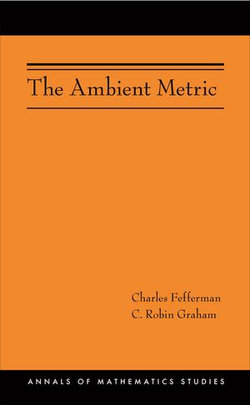# The Ambient Metric (AM-178)

Publication Date: 14/11/2011
\$86.23

This book develops and applies a theory of the ambient metric in conformal geometry. This is a Lorentz metric in n+2 dimensions that encodes a conformal class of metrics in n dimensions. The ambient metric has an alternate incarnation as the Poincaré metric, a metric in n+1 dimensions having the conformal manifold as its conformal infinity. In this realization, the construction has played a central role in the AdS/CFT correspondence in physics.

The existence and uniqueness of the ambient metric at the formal power series level is treated in detail. This includes the derivation of the ambient obstruction tensor and an explicit analysis of the special cases of conformally flat and conformally Einstein spaces. Poincaré metrics are introduced and shown to be equivalent to the ambient formulation. Self-dual Poincaré metrics in four dimensions are considered as a special case, leading to a formal power series proof of LeBrun's collar neighborhood theorem proved originally using twistor methods. Conformal curvature tensors are introduced and their fundamental properties are established. A jet isomorphism theorem is established for conformal geometry, resulting in a representation of the space of jets of conformal structures at a point in terms of conformal curvature tensors. The book concludes with a construction and characterization of scalar conformal invariants in terms of ambient curvature, applying results in parabolic invariant theory.

ISBN:
9781400840588
9781400840588
Category:
Analytic geometry
Format: